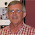## Saturday, September 15, 2012

### Spherically Distributed Errors in Regression Models

Let's think about the standard linear regression model that we encounter in our introductory econometrics courses:

y = Xβ + ε .                      (1)

By writing the model in this form, we've already made two assumptions about the stochastic relationship between the dependent variable, y, and the regressors (the columns of the X matrix). First, the relationship is a parametric one - hence the presence of the coefficient vector, β; and second, the relationship is a linear one. That's to say, the model is linear in these parameters. If it wasn't, we wouldn't be able to write the model in the form given in equation (1).

However, the model isn't fully specified until we lay out any assumptions that are being made about the regressors and the random error term, ε. Now, let's consider the full set of (rather stringent) assumptions that we usually begin with:

1. The X matrix has full column rank, say "k".
2. The columns of X are either non-random (strictly, "fixed in repeated samples"); or if they are random, they are independent of the values of ε.
3. The mean of the distribution that generates the (unobserved) elements of the ε vector is zero.
4. The variance of the distribution that generates each elements of ε is the same (say, σ2). That is, the errors are "homoskedastic".
5. The unobserved values of the ε vector are pair-wise uncorrelated. That is, they don't exhibit any "autocorrelation".
6. The random errors are generated according to a Normal process.
The last of these assumptions can be relaxed in quite a general way without affecting any of the usual results that we typically establish by using it. (See here.)

You'll remember we can combine assumptions 3 to 5, and express them in the form:

ε ~ [0 , σ2In]  ,                    (2)

and we usually describe the statement, (2), by saying that the errors of the model are "spherically distributed".

Students have often asked me where this last this piece of language comes from.

A while back I put together a handout that discusses the notion of "spherically distributed errors", and I'm using it right now with  my introductory graduate econometrics course. Rather than replicate the information in that handout here, you can download the pdf file.

If you use it with your own classes, an acknowledgment would be appreciated.

© 2012, David E. Giles

#### 6 comments:

1.Great images! Thanks, this is a great resource. I've always just said "It looks like a sphere" then tried to draw a picture, but this is far better.

1.Thanks Dan - glad it's helpful.

2.In the last set, do we have a somewhat positive correlation, with Var(y)>Var(x)?

What did you draw these with?

3.That's right. I drew these using the fMultivar package in R. See the next post (16 September) for a link to some R code that does this sort of thing.

4.Thanks! I would like to ask one more question if I may.

I am trying to figure out why I would care about the iso-probability curves being circular. I am thinking of your $x$ and $y$ in a panel-ish context as $\eps_{it}$ and $\eps_{jt}$, and bivariate distribution represents shocks at different times. The expected value of the shocks at each time is zero, but we might have some correlation between units $i$ and $j$. They might be two firms located in the same town, for example.

The peak of the distribution (and innermost iso-probability ellipse) is at (0,0). Suppose I get a negative draw of $\eps_{it}=-1$ (or $x=-1$). In the spherical case, the most likely value of corresponding $\eps_{jt}$ is zero. I can see that by looking at where the vertical line from $x=-1$ intersects the highest probability ellipse. In the correlated case, the same logic say that's no longer true. However, in the case where the shocks are uncorrelated, but one has a higher variance, the first geometric intuition still goes through.

Can you explain where I am going wrong? Is it by equating the expected value with the highest valued iso-probability ring?

5.Dimitriy - I believe your last sentence gives the explanation.